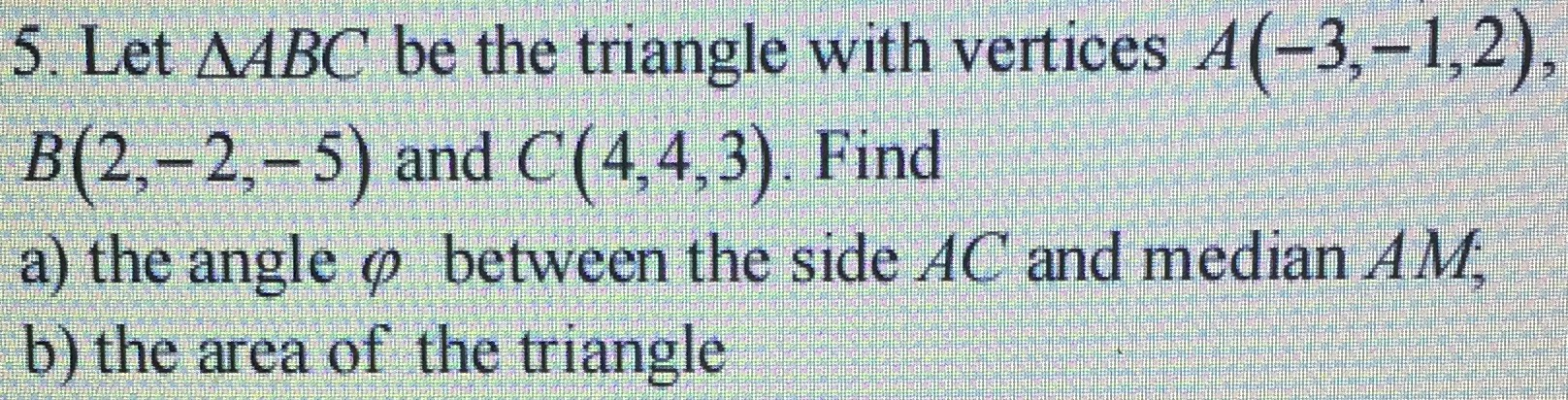### Still have math questions?

Trigonometry
QuestionLet $$\triangle A B C$$ be the triangle with vertices $$A ( - 3 , - 1,2 )$$ ,

$$B ( 2 , - 2 , - 5 )$$ and $$C ( 4,4,3 )$$ . Find

a) the angle $$\varphi$$ between the side $$A C$$ and median $$A M$$ ;

b) the area of the triangle

Angle between AC and Median AM = $$\frac{\angle CAB}{2}= 24.951°$$